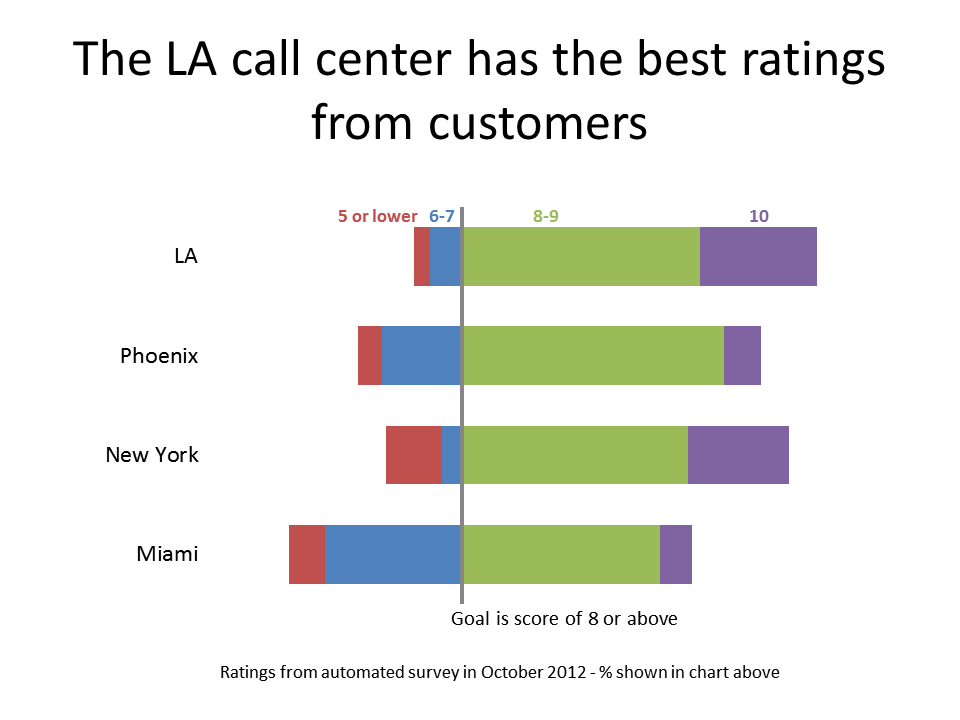# Diverging Stacked Bar Chart Calculator

A diverging stacked bar chart allows you to show two or more segments in multiple category bars compared to a goal value. The segments representing values below the goal value are shown to the left of the goal line, and the segments representing the values above the goal value are shown to the right of the goal line. The segments represent percentages and the total length of the bar for each category is the same. It is a good visual to use when you are trying to emphasize the proportions above the goal value. Here is an example:Each category can represent a different region (as shown in the example above), different products, or different time frames (for example, the proportion above the goal increases each year over four years).

While this looks like a difficult graph to calculate and create, it is actually just a regular stacked bar chart with the values ordered so that they appear in the correct sequence from left to right in each bar. The challenge is to get the values for each bar correct, because the segments to the left of the goal use negative values, and to get the sequence of the values correct, because the sequence is not the same as is calculated with smallest to largest going from left to right. I have created a calculator that does the calculations for you. It is part of my Calculators For Visuals Excel file that you can download.